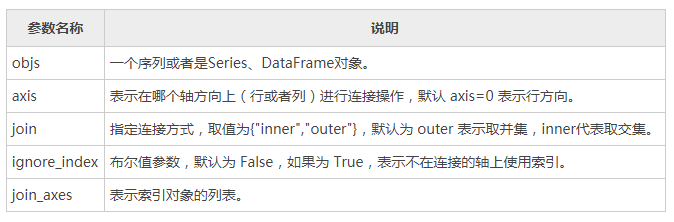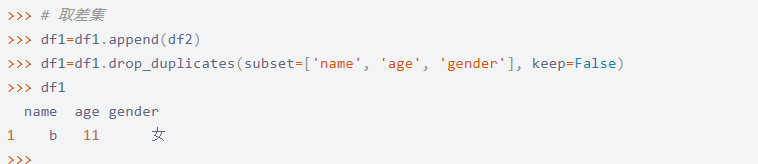# Pandas求两个dataframe差集 详解

1、交集

intersected=pd.merge(df1,df2,how='inner')

2、差集（df1-df2为例）

diff=pd.concat([df1,df2,df2]).drop_duplicates(keep=False)

1、Pandas 通过 concat() 函数能够轻松地将 Series 与 DataFrame 对象组合在一起，函数的语法格式如下： pd.concat(objs,axis=0,join='outer',join_axes=None,ignore_index=False)2、需要对dataframe中的一列值有重复的，应用drop_duplicates解决了此问题。

pd_data=pd.DataFrame(data=data)
print(pd_data)
t=pd_data.drop_duplicates(subset=['c','b'],keep='last',inplace=False)
print(t)

keep='first'表示保留第一次出现的重复行，是默认值。keep另外两个取值为"last"和False，分别表示保留最后一次出现的重复行和去除所有重复行。

inplace=True表示直接在原来的DataFrame上删除重复项，而默认值False表示生成一个副本。如果要生成新的DataFrame:,inplace=False

subset要去重的列。subset=['c','b']，表示行中的记录：c和b列都重复的。

3、将concat和drop_duplicates结合起来就解决了求差集的问题。